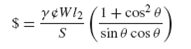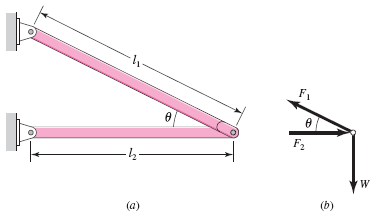### Create an Account

Already have account?

### Forgot Your Password ?

Home / Questions / The engineering designer must create invent the concept and connectivity of the elements t...

# The engineering designer must create invent the concept and connectivity of the elements that constitute a design and not lose sight of the need to develop ideas with optimality in mind A useful

The engineering designer must create (invent) the concept and connectivity of the elements that constitute a design, and not lose sight of the need to develop ideas with optimality in mind. A useful design attribute can be cost, which can be related to the amount of material used (volume or weight). When you think about it, the weight is a function of the geometry and density. When the design is solidified, finding the weight is a straightforward, sometimes tedious task. The figure depicts a simple bracket frame that has supports that project from a wall column. The bracket supports a chain-fall hoist. Pinned joints are used to avoid bending. The cost of a link can be approximated by \$ = ¢Alγ , where ¢ is the cost of the link per unit weight, A is the cross-sectional area of the prismatic link, l is the pin-to-pin link length, and γ is the specific weight of the material used. To be sure, this is approximate because no decisions have been made concerning the geometric form of the links or their fittings. By investigating cost now in this approximate way, one can detect whether a particular set of proportions of the bracket (indexed by angle θ) is advantageous. Is there a preferable angle θ? Show that the cost can be expressed aswhere is the weight of the hoist and load, and is the allowable tensile or compressive stress in the link material (assume = |Fi /A| and no column buckling action). What is the desirable angle θ corresponding to the minimal cost?Problem 1–6

(a) A chain-hoist bracket frame.

(b) Free body of pin.

Jun 24 2020 View more View Less

#### Answer (Solved)Subscribe To Get Solution